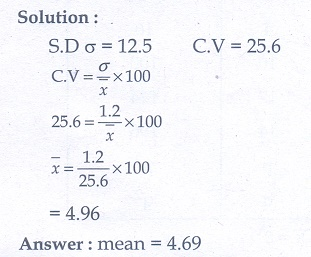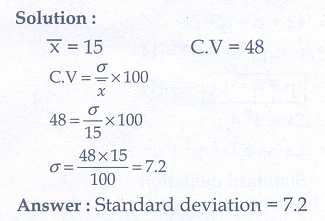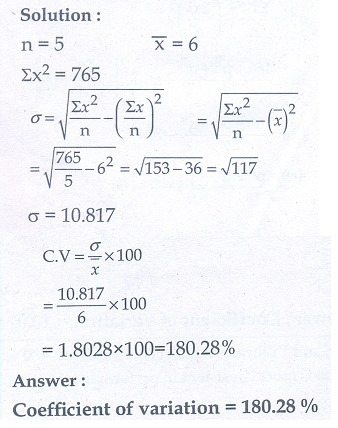Home | | Maths 10th Std | Exercise 8.2: Coefficient of Variation

# Exercise 8.2: Coefficient of Variation

Maths Book back answers and solution for Exercise questions - Mathematics : Statistics And Probability: Coefficient of Variation : Exercise Problem Questions with Answer

Exercise 8.2

1. The standard deviation and mean of a data are 6.5 and 12.5 respectively. Find the coefficient of variation.2. The standard deviation and coefficient of variation of a data are 1.2 and 25.6 respectively. Find the value of mean.3. If the mean and coefficient of variation of a data are 15 and 48 respectively, then find the value of standard deviation.4. If n = 5 ,= 6 , Σx2  = 765 , then calculate the coefficient of variation.5. Find the coefficient of variation of 24, 26, 33, 37, 29, 31.6. The time taken (in minutes) to complete a homework by 8 students in a day are given by 38, 40, 47, 44, 46, 43, 49, 53. Find the coefficient of variation.7. The total marks scored by two students Sathya and Vidhya in 5 subjects are 460 and 480 with standard deviation 4.6 and 2.4 respectively. Who is more consistent in performance?8. The mean and standard deviation of marks obtained by 40 students of a class in three subjects Mathematics, Science and Social Science are given below.Which of the three subjects shows highest  variation  and  which  shows lowest variation in marks?9. The temperature of two cities A and B in a winter season are given below.Find which city is more consistent in temperature changes?1. 52%

2. 4.69

3. 7.2

4. 180.28%

5. 14.4%

6. 10.07%

7.Vidhya

8. Science, Social

9. City A

Tags : Problem Questions with Answer, Solution | Statistics | Mathematics , 10th Mathematics : UNIT 8 : Statistics And Probability
Study Material, Lecturing Notes, Assignment, Reference, Wiki description explanation, brief detail
10th Mathematics : UNIT 8 : Statistics And Probability : Exercise 8.2: Coefficient of Variation | Problem Questions with Answer, Solution | Statistics | Mathematics